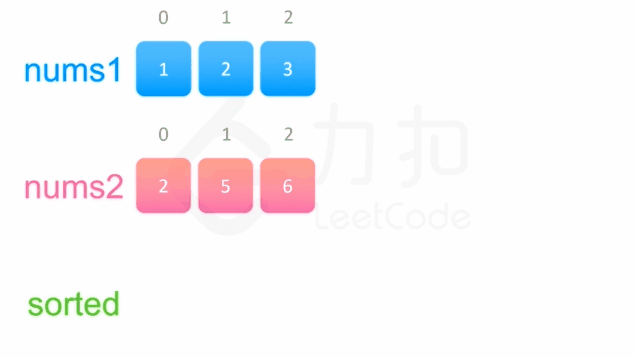# LeetCode-三周攻克数据结构-数组篇-12021 年 08 月 01 日

## Day1

### 1. 存在重复元素

``````示例 1:

#### 方法1：Set结构

``````function containsDuplicate(nums: number[]): boolean {
const setNums: Array<any> = [...new Set(nums)]
return setNums.length !== nums.length
};``````

#### 方法2：优化版暴力解法

``````function containsDuplicate(nums: number[]): boolean {
let newArr: Array<any> = [...nums]
let res: boolean = false
for(let i = 0; i <nums.length; i++){
const item: any = newArr.splice(0, 1)
res = newArr.includes(item)
if(res)break
}
return res
};``````

### 2. 最大子序和

``````示例 1：

#### 方法1：动态规划

``````function maxSubArray(nums: number[]): number {
let sum = 0;
let ans = nums
for(const num of nums) {
if(sum > 0) {
sum += num
} else {
sum = num
}
ans = Math.max(ans, sum)

}
return ans
};``````

## DAY2

### 1. 两数之和

``````给定一个整数数组 nums 和一个整数目标值 target，请你在该数组中找出 和为目标值 target  的那 两个 整数，并返回它们的数组下标。

#### 方法1：爆破

2 个 for 循环直接暴力解法

``````  function twoSum(nums: number[], target: number): number[] {
for (let i = 0; i < nums.length - 1; i++) {
for (let j = i + 1; j < nums.length; j++) {
if (target === nums[j] + nums[i]) {
return [i, j];
}
}
}
}``````

#### 方法2：哈希表查询

1. 建立一个`值``下标`的哈希表
2. 遍历一次数组用 `target - 数组的value` = 结果，结果在我们的哈希表里面执行 `.has`找得到就返回下标
``````
function twoSum(nums: number[], target: number): number[] {
let map = new Map<number, number>()
for (let i = 0; i < nums.length; i++) {
if(map.has(target - nums[i])){
// 返回建立的哈希表的下标的值，和当前的下标
return [map.get(target - nums[i]), i];
} else {
// 储存值为 key 下标为 index
map.set(nums[i], i);
}
}
return []
}``````

### 2. 合并两个有序数组

#### 方法1：合并数组排序

``````function merge(nums1: number[], m: number, nums2: number[], n: number): void {
nums1.splice(m, nums1.length - m, ...nums2);
nums1.sort((a, b) => a - b);
};``````

#### 方法2：双指针``````/**
Do not return anything, modify nums1 in-place instead.
*/
function merge(nums1: number[], m: number, nums2: number[], n: number): void {
// 定义2个从0 开始的指针，分别用来遍历 nums1 和 nums2
let p1 = 0, p2 = 0;
// 空数组储存排序后的值
const sorted = new Array(m + n).fill(0);
// 当次排序的值
var cur: number;

// 开始遍历2个数组
while (p1 < m || p2 < n) {
if (p1 === m) {
cur = nums2[p2++];
} else if (p2 === n) {
cur = nums1[p1++];
} else if (nums1[p1] < nums2[p2]) {
cur = nums1[p1++];
} else {
cur = nums2[p2++];
}

sorted[p1 + p2 - 1] = cur;
}
for (let i = 0; i != m + n; ++i) {
nums1[i] = sorted[i];
}
};``````

#### 方法3：逆向双指针

``````/**
Do not return anything, modify nums1 in-place instead.
*/
function merge(nums1: number[], m: number, nums2: number[], n: number): void {
// 指针从尾部开始
let p1 = m - 1
let p2 = n - 1
// 总长度
let tail = m + n - 1;
// 当次排序的值
let cur: number;

while (p1 >= 0 || p2 >= 0) {
if (p1 === -1) {
cur = nums2[p2--];
} else if (p2 === -1) {
cur = nums1[p1--];
} else if (nums1[p1] > nums2[p2]) {
cur = nums1[p1--];
} else {
cur = nums2[p2--];
}
nums1[tail--] = cur;
}
};``````

• 小尾巴~